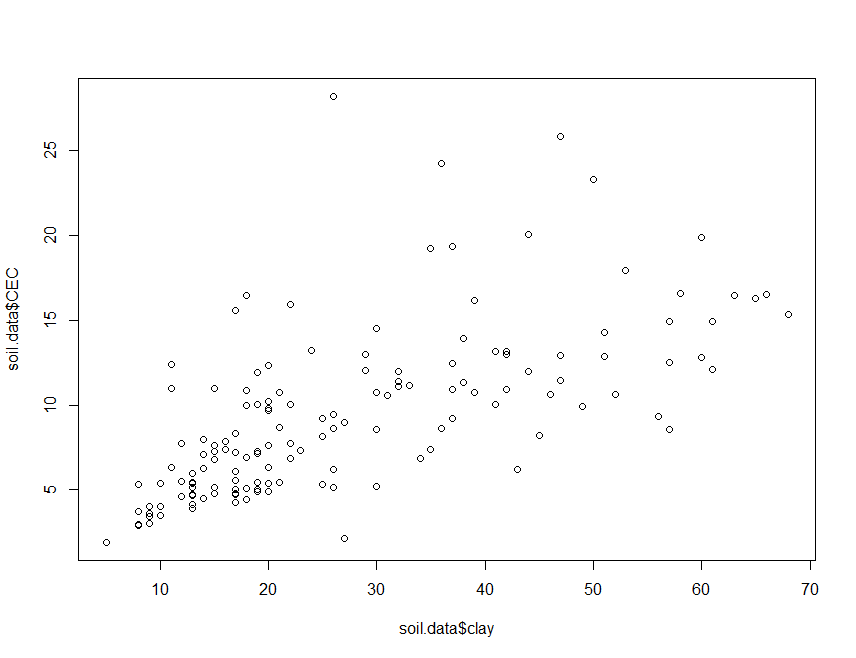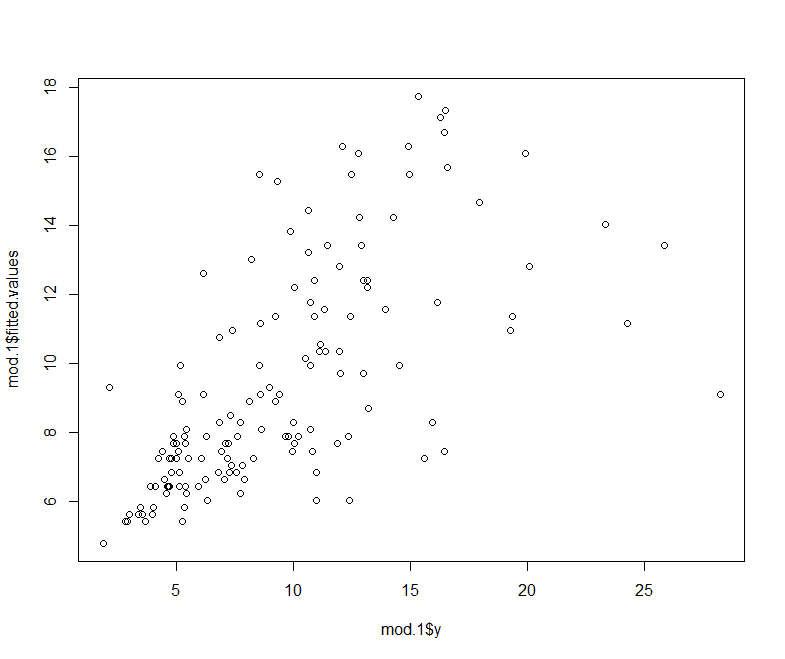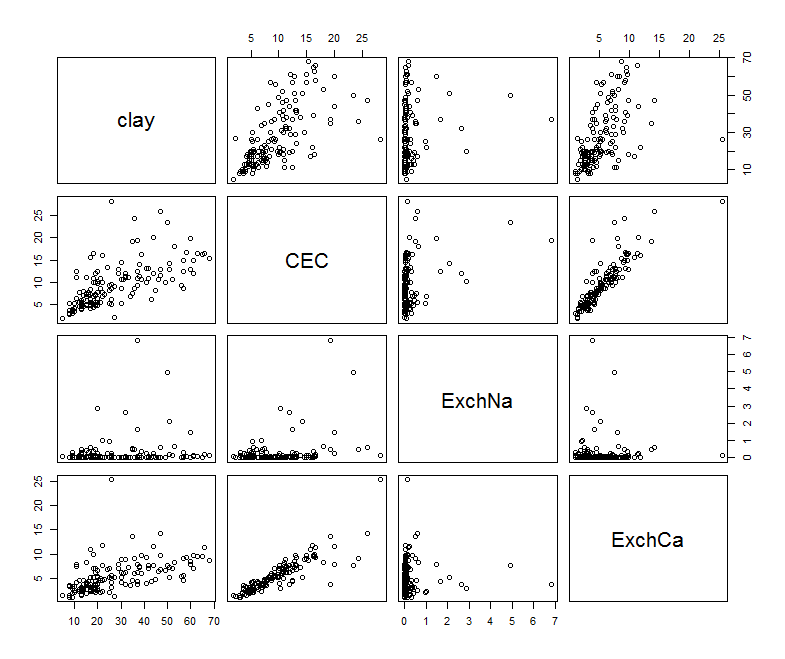#### CODE:

Get the code used for this section here

## Linear models: the basics

### The lm function, model formulas, and statistical output

In R several classical statistical models can be implemented using one function: lm (for linear model). The lm function can be used for simple and multiple linear regression, analysis of variance (ANVOVA), and analysis of covariance (ANCOVA). The help file for lm lists the following.

lm(formula, data, subset, weights, na.action, method = qr'', model = TRUE, x = FALSE, y = FALSE, qr = TRUE, singular.ok = TRUE, contrasts = NULL, offset, ...)

The first argument in the lm function call (formula) is where you specify the structure of the statistical model. This approach is used in other R functions as well, such as glm, gam, and others, which we will later investigate. The most common structures of a statistical model are:

y ∼ x :Simple linear regression of y on x

y ∼ x + z :Multiple regression of y on x and z

There are many others, but it is these model structures that are used in one form or another for digital soil mapping.

There is some similarity between the statistical output in R and in other statistical software programs. However, by default, R usually gives only basic output. More detailed output can be retrieved with the summary function. For specific statistics, you can use extractor functions, such as coef or deviance. Output from the lm function is of the class lm, and both default output and specialized output from extractor functions can be assigned to objects (this is of course true for other model objects as well). This quality is very handy when writing code that uses the results of statistical models in further calculations or in compiling summaries.

### Linear regression

To demonstrate simple linear regression in R, we will again use the soil.data set. Here we will regress CEC content on clay.

    library(ithir)
data(USYD_soil1)
soil.data <- USYD_soil1
summary(cbind(clay = soil.data$clay, CEC = soil.data$CEC))

##       clay            CEC
##  Min.   : 5.00   Min.   : 1.900
##  1st Qu.:15.00   1st Qu.: 5.350
##  Median :21.00   Median : 8.600
##  Mean   :26.95   Mean   : 9.515
##  3rd Qu.:37.00   3rd Qu.:12.110
##  Max.   :68.00   Max.   :28.210
##  NA's   :17      NA's   :5


The (alternative) hypothesis here is that clay content is a good predictor of CEC. As a start, let us have a look at what the data looks like.

    plot(soil.data$clay, soil.data$CEC)plot of against from the soil.data set.

There appears to be some meaningful relationship. To fit a linear model, we can use the lm function:

    mod.1 <- lm(CEC ~ clay, data = soil.data, y = TRUE, x = TRUE)
mod.1

##
## Call:
## lm(formula = CEC ~ clay, data = soil.data, x = TRUE, y = TRUE)
##
## Coefficients:
## (Intercept)         clay
##      3.7791       0.2053


R returns only the call and coefficients by default. You can get more information using the summary function.

    summary(mod.1)

##
## Call:
## lm(formula = CEC ~ clay, data = soil.data, x = TRUE, y = TRUE)
##
## Residuals:
##     Min      1Q  Median      3Q     Max
## -7.1829 -2.3369 -0.6767  1.0185 19.0924
##
## Coefficients:
##             Estimate Std. Error t value Pr(>|t|)
## (Intercept)  3.77913    0.63060   5.993 1.58e-08 ***
## clay         0.20533    0.02005  10.240  < 2e-16 ***
## ---
## Signif. codes:  0 '***' 0.001 '**' 0.01 '*' 0.05 '.' 0.1 ' ' 1
##
## Residual standard error: 3.783 on 144 degrees of freedom
##   (20 observations deleted due to missingness)
## Multiple R-squared:  0.4214, Adjusted R-squared:  0.4174
## F-statistic: 104.9 on 1 and 144 DF,  p-value: < 2.2e-16


Clay does appear to be a significant predictor of CEC as one would generally surmise when applying our soil science knowledge. As mentioned above, the output from the lm function is an object of class lm. These objects are lists that contain at least the following elements (you can find this list in the help file for lm):

• coefficients: a named vector of model coefficients
• residuals: model residuals (observed - predicted values)
• fitted.value: the fitted mean values
• rank: a numeric rank of the fitted linear model
• weights: (only for weighted fits) the specified weights
• df.residual: the residual degrees of freedom
• call: the matched call
• terms: the terms object used
• contrasts: (only where relevant) the contrasts used
• xlevels: (only where relevant) a record of the levels of the factors used in fitting
• offset: the offset used (missing if none were used)
• y: if requested, the response used
• x: if requested, the model matrix used
• model: if requested (the default), the model frame used
• na.action: (where relevant) information returned by model frame on the handling of NAs

To get at the elements listed above, you can simply index the lm object, i.e. call up part of the list.

    mod.1$coefficients ## (Intercept) clay ## 3.7791256 0.2053256  However, R has several extractor functions designed precisely for pulling data out of statistical model output. Some of the most commonly used ones are: add1, alias, anova, coef, deviance, drop1, effects, family, formula, kappa, labels, plot, predict, print, proj, residuals, step, summary, and vcov. For example:  coef(mod.1) ## (Intercept) clay ## 3.7791256 0.2053256 head(residuals(mod.1)) ## 1 2 3 4 6 7 ## -0.1317300 -1.7217300 -2.5617300 -2.5017300 -0.5226822 -2.0370556  As mentioned before, the summary function is a generic function. What it does and what it returns is dependent on the class of its first argument. Here is a list of what is available from the summary function for this model:  names(summary(mod.1)) ##  "call" "terms" "residuals" "coefficients" ##  "aliased" "sigma" "df" "r.squared" ##  "adj.r.squared" "fstatistic" "cov.unscaled" "na.action"  To extract some of the information in the summary which is of a list structure, we would use:  summary(mod.1)[] ## Estimate Std. Error t value Pr(>|t|) ## (Intercept) 3.7791256 0.63060292 5.992877 1.577664e-08 ## clay 0.2053256 0.02005048 10.240431 7.910414e-19  To look at the statistical summary. What is the R2 of mod.1? Answer:  summary(mod.1)[["r.squared"]] ##  0.4213764  This flexibility is useful, but makes for some redundancy in R. For many model statistics, there are three ways to get your data: an extractor function (such as coef), indexing the lm object, and indexing the summary function. The best approach is to use an extractor function whenever you can. In some cases, the summary function will return results that you can not get by indexing or using extractor functions. Once we have fit a model in R, we can generate predicted values using the predict function.  head(predict(mod.1)) ## 1 2 3 4 6 7 ## 5.421730 5.421730 5.421730 5.421730 15.482682 5.627056  Lets plot the observed vs. the predict from this model.  plot(mod.1$y, mod.1\$fitted.values)observed vs. predicted plot of CEC from soil.data.

As we will see later on, the predict function works for a whole range of statistical models in R. Not just lm objects. We can treat the predictions as we would any vector. For example we can add them to the above plot or put them back in the original data frame. The predict function can also give confidence and prediction intervals.

    head(predict(mod.1, int = "conf"))

##         fit       lwr       upr
## 1  5.421730  4.437845  6.405615
## 2  5.421730  4.437845  6.405615
## 3  5.421730  4.437845  6.405615
## 4  5.421730  4.437845  6.405615
## 6 15.482682 14.152940 16.812425
## 7  5.627056  4.673657  6.580454


A quick way to demonstrate multiple linear regression in R, we will regress CEC on clay plus the exchangeable cations: ExchNa and ExchCa. First lets subset these data out, then get their summary statistics.

    subs.soil.data <- soil.data[, c("clay", "CEC", "ExchNa", "ExchCa")]
summary(subs.soil.data)

##       clay            CEC             ExchNa           ExchCa
##  Min.   : 5.00   Min.   : 1.900   Min.   :0.0000   Min.   : 1.010
##  1st Qu.:15.00   1st Qu.: 5.350   1st Qu.:0.0200   1st Qu.: 2.920
##  Median :21.00   Median : 8.600   Median :0.0500   Median : 4.610
##  Mean   :26.95   Mean   : 9.515   Mean   :0.2563   Mean   : 5.353
##  3rd Qu.:37.00   3rd Qu.:12.110   3rd Qu.:0.1500   3rd Qu.: 7.240
##  Max.   :68.00   Max.   :28.210   Max.   :6.8100   Max.   :25.390
##  NA's   :17      NA's   :5        NA's   :5        NA's   :5


A quick way to look for relationships between variables in a data frame is with the cor function. Note the use of the na.omit function.

    cor(na.omit(subs.soil.data))

##             clay       CEC      ExchNa      ExchCa
## clay   1.0000000 0.6491351  0.17104962  0.54615778
## CEC    0.6491351 1.0000000  0.33369789  0.87570029
## ExchNa 0.1710496 0.3336979  1.00000000 -0.02055496
## ExchCa 0.5461578 0.8757003 -0.02055496  1.00000000


To visualize these relationships, we can use pairs.

    pairs(na.omit(subs.soil.data))A pairs plot of a select few soil attributes from the soil.data set.

There are some interesting relationships here. Now for fitting the model:

    mod.2 <- lm(CEC ~ clay + ExchNa + ExchCa, data = subs.soil.data)
summary(mod.2)

##
## Call:
## lm(formula = CEC ~ clay + ExchNa + ExchCa, data = subs.soil.data)
##
## Residuals:
##     Min      1Q  Median      3Q     Max
## -5.2008 -0.7065 -0.0470  0.6455  9.4025
##
## Coefficients:
##             Estimate Std. Error t value Pr(>|t|)
## (Intercept) 1.048318   0.274264   3.822 0.000197 ***
## clay        0.050503   0.009867   5.119 9.83e-07 ***
## ExchNa      2.018149   0.163436  12.348  < 2e-16 ***
## ExchCa      1.214156   0.046940  25.866  < 2e-16 ***
## ---
## Signif. codes:  0 '***' 0.001 '**' 0.01 '*' 0.05 '.' 0.1 ' ' 1
##
## Residual standard error: 1.522 on 142 degrees of freedom
##   (20 observations deleted due to missingness)
## Multiple R-squared:  0.9076, Adjusted R-squared:  0.9057
## F-statistic: 465.1 on 3 and 142 DF,  p-value: < 2.2e-16


For much of the remainder of the exercises, we will be investigating these regression type relationships using a variety of different model types for soil spatial prediction. These fundamental modelling concepts of the lm will become useful as we progress ahead.

### Exercises

1. Using the soil.data set firstly generate a correlation matrix of all the soil variables.

2. Choose two variables that you think would be good to regress against each other, and fit a model. Is the model any good? Can you plot the observed vs. predicted values? Can you draw a line of concordance (maybe want to consult an appropriate help file to do this). Something a bit trickier: Can you add the predictions to the data frame soil.data correctly.

3. Now repeat what you did for the previous question, except this time perform a multiple linear regression i.e. use more than 1 predictor variable to make a prediction of the variable you are targeting.

Updated on